Measuring Focal Length
Prepared 2005-11-15 by Bill Claff

### Introduction

Extension () (as in bellows or extension tubes), magnification (), and focal length () are interrelated as follows:WhereAnd the valuesand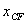are the magnification and extension of the lens at the current focus setting of the lens.
Note that lens extension due to focusing can be internal and the value ofis never positive.

Typically, when the current focus setting is infinity,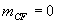, and. So, and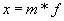.

### Application

At infinity focus a minimum of one magnification measurement at a non‑zero extension is required to determine focal length.

At focus settings other than infinity a minimum of two magnification measurements at different non‑zero extensions are required to determine focal length and extension of the lens.

In practice, even with precise measurements, multiple data points are required to obtain satisfactory results.

Because extension is known and magnification is measured we take our data points and do a linear least squares fit to: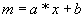Where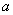andare the slope and intercept from out least squares fit.

ThenandIn practice, slight positive values forat infinity focus may arise due to imprecise data and because due to mechanical tolerances and lens design the lens may in fact focus slightly beyond infinity.

This analysis can be performed on a lens that is reverse mounted and for many lenses this data is easier to collect.

For a reverse mounted lens keep in mind that the value ofis relative to where the lens attaches to the camera body, adapter ring, or extension tube.

### Field Of View

Naturally, once we have a more precise measurement of focal length we also have a more precise measurement of field of view.

We can calculate focal length and field of view for any focus setting regardless of whether an extension ring is present.
Application to panoramic corrections for a lens set to a hyper‑focal distance rather than infinity would be a good example.

### A Final Note

In cases where I have collected both normal mounted and reverse mounted data the computed reverse mounted focal lengths have been slightly less than the computed normal focal lengths.
These differences are less than 1mm. I have no explanation for this phenomenon.